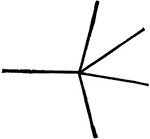360° Sum of Angles

Illustration showing that the sum of all the angles about a point equals 360°.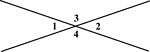Vertical Angles

Illustration showing that angles 1 and 2 are vertical and angles 3 and 4 are vertical.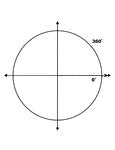360° and 0° Coterminal Angles

Illustration showing coterminal angles of 360° and 0°. Coterminal angles are angles drawn in…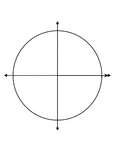360° and 0° Coterminal Angles

Illustration showing coterminal angles of 360° and 0°. Coterminal angles are angles drawn in…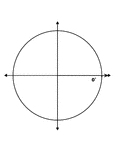360° and 0° Coterminal Angles

Illustration showing coterminal angles of 360° and 0°. Coterminal angles are angles drawn in…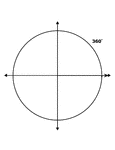360° and 0° Coterminal Angles

Illustration showing coterminal angles of 360° and 0°. Coterminal angles are angles drawn in…